Mona A.Ashok Nagar, Bangalore, India - 560025Featured

Mona A.Experienced Maths Teacher

Ashok Nagar, Bangalore, India - 560025.

1 Student taught

5.0

UrbanPro Rating

Details verified of Mona A.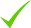IdentityEducation

Know how UrbanPro verifies Tutor details

Identity is verified based on matching the details uploaded by the Tutor with government databases.

Overview

1. Have taught Mathematics in schools in Delhi and Indore.
2. Bachelor's degree in Mathematical Statistics from Lady Shri Ram college, Delhi
3. Masters degree in Operational Research, Delhi University.
4. PG diploma in Computer Science , NIIT, Delhi
5. Have given personalised coaching for class 12 and GRE as well.

Languages Spoken

Hindi Mother Tongue (Native)

English Proficient

Education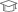Lady Shri Ram College, Delhi University 1989

Bachelor of Arts (honours) Mathematical StatisticsDelhi University 1992

M.A. Operational Research

Ashok Nagar, Bangalore, India - 560025

Verified Info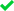ID VerifiedEducation VerifiedPhone VerifiedEmail Verified

Demo Class

Yes, not charged

Report this Profile

Is this listing inaccurate or duplicate? Any other problem?

Please tell us about the problem and we will fix it.

Please describe the problem that you see in this page.

Type the letters as shown below *

Please enter the letters as show below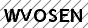Class 10 Tuition Overview

Class 10 Tuition

Class Location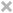Student's HomeTutor's HomeOnline (video chat via skype, google hangout etc)

Years of Experience in Class 10 Tuition

5

Board

CBSE

CBSE Subjects taught

Mathematics

Experience in School or College

St. Xavier's school, Delhi Army Public School, Delhi Indore Public School, Delhi

Taught in School or College

Yes

Reviews (1)

this is test message this is test message this is test message this is test message this is test message this is test message this is test message

5.0 out of 5.0 1 review

5.0051
S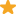"Can't get a better teacher than her. She won't help you to just get the answers to the problems but also help in getting the basics right. Her understanding of child psychology is beyond compare. I am recommending her for not just for class 9 but for all classes from 7 - 10. At least have one session with her and you will know what I am talking about. She is awesome! "

Have you attended any class with Mona? Write a Review

FAQs

1. Which school boards of Class 10 do you teach for?

CBSE

2. Do you have any prior teaching experience?

Yes

3. Which classes do you teach?

I teach Class 10 Tuition, Class 7 Tuition, Class 8 Tuition and Class 9 Tuition Classes.

4. Do you provide a demo class?

Yes, I provide a free demo class.

5. How many years of experience do you have?

I have been teaching for 5 years.

Answers by Mona (9)

Answered on 26 Aug CBSE/Class 9/Mathematics/Surface Area and Volumes/NCERT Solutions/Exercise 13.7

V = (pi*r*r*h)/3 = (3.14*5*5*12)/3 = 3.14*100 =314 cu.cm
Dislike Bookmark

Answered on 13 Aug CBSE/Class 9/Mathematics/Surface Area and Volumes/NCERT Solutions/Exercise 13.7

V = πr²h/3 1570 = 3.14*r²*15/3 1570 = 15.7r² r² = 100 r = 10 cm
Dislike Bookmark

Answered on 13 Aug CBSE/Class 9/Mathematics/Surface Area and Volumes/NCERT Solutions/Exercise 13.7

V = πr²h/3 48π = 9πr²/3 = 3πr² 48/3 = 16 = r² r = 4 cm diameter = 2r = 8cm
Dislike Bookmark

Answered on 12 Aug CBSE/Class 9/Mathematics/Surface Area and Volumes/NCERT Solutions/Exercise 13.7

V = (1/3)*π*r*r*h r =10.5/2= 5.25 V= (1/3)*(22/7)*5.25*5.25*3 = 86.625 m^3 Area of the canvas = curved surface of heap = π*r*l l = √(r*r+h*h) = √(36.5625) =6.046 m (approx) πrl = 3.14*5.25*6.046 = 99.668 m^2 ...more

V = (1/3)*π*r*r*h

r =10.5/2= 5.25

V= (1/3)*(22/7)*5.25*5.25*3

= 86.625 m^3

Area of the canvas = curved surface of heap = π*r*l

l = √(r*r+h*h) = √(36.5625)

=6.046 m (approx)

πrl = 3.14*5.25*6.046

= 99.668 m^2

Dislike Bookmark

Answered on 12 Aug CBSE/Class 9/Mathematics/Surface Area and Volumes/NCERT Solutions/Exercise 13.7

(i)V = 1/3 * π* r*r*h 9856 = 1/3 *(22/7) *14*14*h h= (9856*3*7)/(22*14*14) =48 cm (ii) slant height l = √(r*r + h*h) = √ (196+2304) = √2500 = 50 cm (iii) curved surface area π*r*l = (22/7)*14*50 =2200 cm^2 ...more

(i)V = 1/3 * π* r*r*h

9856 = 1/3 *(22/7) *14*14*h

h=  (9856*3*7)/(22*14*14)

=48 cm

(ii) slant height

l = √(r*r + h*h) = √ (196+2304)

= √2500 = 50 cm

(iii) curved surface area

π*r*l = (22/7)*14*50

=2200 cm^2

Dislike Bookmark

x
Class 10 Tuition 5.0

Class LocationStudent's HomeTutor's HomeOnline (video chat via skype, google hangout etc)

Years of Experience in Class 10 Tuition

5

Board

CBSE

CBSE Subjects taught

Mathematics

Experience in School or College

St. Xavier's school, Delhi Army Public School, Delhi Indore Public School, Delhi

Taught in School or College

Yes

Class 9 Tuition 5.0

Class LocationStudent's HomeTutor's HomeOnline (video chat via skype, google hangout etc)

Years of Experience in Class 9 Tuition

5

Board

CBSE

CBSE Subjects taught

Mathematics

Experience in School or College

St Xavier's school , Delhi Army Public School, Delhi Indore Public School, Indore

Taught in School or College

Yes

Class 8 Tuition 5.0

Class LocationStudent's HomeTutor's HomeOnline (video chat via skype, google hangout etc)

Years of Experience in Class 8 Tuition

20

Board

ICSE, CBSE

CBSE Subjects taught

Mathematics

ICSE Subjects taught

Mathematics

Experience in School or College

St Xavier's school Delhi Army Public School, Delhi Indore Public School, Indore

Taught in School or College

Yes

Teaching Experience in detail in Class 8 Tuition

Have taught in St Xavier's Delhi and Indore Public School, Indore.

Class 7 Tuition 5.0

Class LocationStudent's HomeTutor's HomeOnline (video chat via skype, google hangout etc)

Years of Experience in Class 7 Tuition

20

Board

ICSE, CBSE

CBSE Subjects taught

Mathematics

ICSE Subjects taught

Mathematics

Experience in School or College

St Xavier's school Delhi Army Public School, Delhi Indore Public School, Indore

Taught in School or College

Yes

Teaching Experience in detail in Class 7 Tuition

St Xavier's school Delhi Indore Public School Indore

this is test message this is test message this is test message this is test message this is test message this is test message this is test message

5.0 out of 5.0 1 review

S"Can't get a better teacher than her. She won't help you to just get the answers to the problems but also help in getting the basics right. Her understanding of child psychology is beyond compare. I am recommending her for not just for class 9 but for all classes from 7 - 10. At least have one session with her and you will know what I am talking about. She is awesome! "

Have you attended any class with Mona? Write a Review

Answers by Mona (9)

Answered on 26 Aug CBSE/Class 9/Mathematics/Surface Area and Volumes/NCERT Solutions/Exercise 13.7

V = (pi*r*r*h)/3 = (3.14*5*5*12)/3 = 3.14*100 =314 cu.cm
Dislike Bookmark

Answered on 13 Aug CBSE/Class 9/Mathematics/Surface Area and Volumes/NCERT Solutions/Exercise 13.7

V = πr²h/3 1570 = 3.14*r²*15/3 1570 = 15.7r² r² = 100 r = 10 cm
Dislike Bookmark

Answered on 13 Aug CBSE/Class 9/Mathematics/Surface Area and Volumes/NCERT Solutions/Exercise 13.7

V = πr²h/3 48π = 9πr²/3 = 3πr² 48/3 = 16 = r² r = 4 cm diameter = 2r = 8cm
Dislike Bookmark

Answered on 12 Aug CBSE/Class 9/Mathematics/Surface Area and Volumes/NCERT Solutions/Exercise 13.7

V = (1/3)*π*r*r*h r =10.5/2= 5.25 V= (1/3)*(22/7)*5.25*5.25*3 = 86.625 m^3 Area of the canvas = curved surface of heap = π*r*l l = √(r*r+h*h) = √(36.5625) =6.046 m (approx) πrl = 3.14*5.25*6.046 = 99.668 m^2 ...more

V = (1/3)*π*r*r*h

r =10.5/2= 5.25

V= (1/3)*(22/7)*5.25*5.25*3

= 86.625 m^3

Area of the canvas = curved surface of heap = π*r*l

l = √(r*r+h*h) = √(36.5625)

=6.046 m (approx)

πrl = 3.14*5.25*6.046

= 99.668 m^2

Dislike Bookmark

Answered on 12 Aug CBSE/Class 9/Mathematics/Surface Area and Volumes/NCERT Solutions/Exercise 13.7

(i)V = 1/3 * π* r*r*h 9856 = 1/3 *(22/7) *14*14*h h= (9856*3*7)/(22*14*14) =48 cm (ii) slant height l = √(r*r + h*h) = √ (196+2304) = √2500 = 50 cm (iii) curved surface area π*r*l = (22/7)*14*50 =2200 cm^2 ...more

(i)V = 1/3 * π* r*r*h

9856 = 1/3 *(22/7) *14*14*h

h=  (9856*3*7)/(22*14*14)

=48 cm

(ii) slant height

l = √(r*r + h*h) = √ (196+2304)

= √2500 = 50 cm

(iii) curved surface area

π*r*l = (22/7)*14*50

=2200 cm^2

Dislike Bookmark

Mona A. describes herself as Experienced Maths Teacher. She conducts classes in Class 10 Tuition, Class 7 Tuition and Class 8 Tuition. Mona is located in Ashok Nagar, Bangalore. Mona takes Online Classes- via online medium. She has 20 years of teaching experience . Mona has completed Bachelor of Arts (honours) Mathematical Statistics from Lady Shri Ram College, Delhi University in 1989 and M.A. Operational Research from Delhi University in 1992. She is well versed in English and Hindi. Mona has got 1 reviews till now with 100% positive feedback.

Recommended Profiles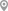Ashok Nagar, Patna SadarAshok Nagar, HyderabadAshok Nagar, RanchiAshok Nagar, Patna SadarAshok Nagar, Patna SadarAshok Nagar, Kolkata

X

Post your Learning Need

Let us shortlist and give the best tutors and institutes.

or

Send Enquiry to Mona

Let Mona know you are interested in their class

Reply to 's review